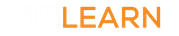### Mathematics Virtual Lessons

### Number A

• Multiplication and Division
• Decimal Calculations
• Negative Number Calculations
• Properties of Numbers
• Order of Operations
• HCF, LCM and Prime Factors
• Rounding
• Estimating
• Limits of Accuracy

### Number B

• Multiply and Divide Fractions
• Fractions and Decimals
• Introduction to Percentages
• Powers and Roots
• Calculate with Powers and Roots
• Standard Form
• Standard Form - Calculations
• Negative and Fractional Powers
• Surds
• Calculate with Surds

### Algebra A

• Substitution
• Simplifying Expressions
• Expanding Brackets
• Expanding two or more brackets
• Factorising Expressions 1
• Factorising Expressions 2
• Rearranging Formula
• Generating Sequences
• Finding the Nth term of sequences

### Algebra B

• Linear graphs 2
• Linear graphs 3
• Other Graphs 1
• Other Graphs 2
• Real life graphs 1
• Real life graphs 2

### Algebra C

• Algebraic Fractions
• Iteration and Functions
• Simultaneous equations 1
• Simultaneous equations 2
• Inequalities
• Solving problems - Algebra
• Algebraic Proof

### Ratio, Proportion and Rates

• Compound units of measure
• Scale drawings and bearings
• Introduction to Ratio
• Dividing by a Ratio
• Ratio Problems 1
• Ratio Problems 2
• Direct and Inverse Proportion 1
• Direct and Inverse Proportion 2
• Percentage of Amounts
• Percentage increase and decrease
• Percentage Change
• Reverse Percentages
• Growth and decay

### Geometry and Measures A

• Angles 2
• Angles 3
• Perimeter and Area
• Area and circumference - Circles
• Sectors
• 3D shapes
• Plans and Elevations
• Surface area
• Surface Area 2
• Volume
• Frustums

### Geometry and Measures B

• Area and volume of similar shapes
• Transformations 1
• Transformations 2
• Constructions
• Loci
• Pythagoras' Theorem

### Geometry and Measures C

• Trigonometry 2
• 3D Pythagoras and Trigonometry
• Trigonometry non-calculator
• Sine and Cosine Rules 1
• Sine and Cosine Rules 2
• Circle theorems 1
• Applying Circle Theorems
• Vectors

### Probability

• Experimental probability and expectation
• Combined events 1
• Combined events 2
• Venn Diagrams

### Statistics

• Introduction to Statistics 2
• Pie charts
• Scatter diagrams
• The Averages
• Frequency Tables and the Averages
• Cumulative frequency
• Histograms